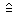# U+2259 ESTIMATES

U+2259 was added to Unicode in version 1.1 (1993). It belongs to the blockMathematical Operators in the Basic Multilingual Plane.

This character is a Math Symbol and is commonly used, that is, in no specific script. The character is also known as corresponds to.

The glyph is not a composition. It has a Neutral East Asian Width. In bidirectional context it acts as Other Neutral and is not mirrored. The glyph can, under circumstances, be confused with 1 other glyphs. In text U+2259 behaves as Alphabetic regarding line breaks. It has type Other for sentence and Other for word breaks. The Grapheme Cluster Break is Any.

The Wikipedia has the following information about this codepoint:

In mathematics and mathematical economics, correspondence is a term with several related but distinct meanings.

• In general mathematics, a correspondence is an ordered triple (X,Y,R), where R is a relation from X to Y, i.e. any subset of the Cartesian product X×Y.

• In algebraic geometry, a correspondence between algebraic varieties V and W is in the same fashion a subset R of V×W, which is in addition required to be closed in the Zariski topology. It therefore means any relation that is defined by algebraic equations. There are some important examples, even when V and W are algebraic curves: for example the Hecke operators of modular form theory may be considered as correspondences of modular curves.
However, the definition of a correspondence in algebraic geometry is not completely standard. For instance, Fulton, in his book on Intersection theory, uses the definition above. In literature, however, a correspondence from a variety X to a variety Y is often taken to be a subset Z of X×Y such that Z is finite and surjective over each component of X. Note the asymmetry in this latter definition; which talks about a correspondence from X to Y rather than a correspondence between X and Y. The typical example of the latter kind of correspondence is the graph of a function f:XY. Correspondences also play an important role in the construction of motives.
• In category theory, a correspondence from to is a functor . It is the "opposite" of a profunctor.
• One-to-one correspondence is an alternate name for a bijection.
• In von Neumann algebra theory, a correspondence is a synonym for a von Neumann algebra bimodule.
• In economics, a correspondence between two sets A and B is a map f:AP(B) from the elements of the set A to the power set of B. This is similar to a correspondence as defined in general mathematics (i.e., a relation,) except that the range is over sets instead of elements. However, there is usually the additional property that for all a in A, f(a) is not empty. In other words, each element in A maps to a non-empty subset of B; or in terms of a relation R as subset of A×B, R projects to A surjectively. A correspondence with this additional property is thought of as the generalization of a function, rather than as a special case of a relation, and is referred to in other contexts as a multivalued function.
An example of a correspondence in this sense is the best response correspondence in game theory, which gives the optimal action for a player as a function of the strategies of all other players. If there is always a unique best action given what the other players are doing, then this is a function. If for some opponent's strategy, there is a set of best responses that are equally good, then this is a correspondence.

## Representations

System Representation
8793
UTF-8 E2 89 99
UTF-16 22 59
UTF-32 00 00 22 59
URL-Quoted %E2%89%99
HTML-Escape &#x2259;
Wrong windows-1252 Mojibake â
HTML-Escape &wedgeq;
alias corresponds to
LaTeX \estimates

## Complete Record

Property Value
Age (age) 1.1
Unicode Name (na) ESTIMATES
Unicode 1 Name (na1)
Block (blk) Math_Operators
General Category (gc) Math Symbol
Script (sc) Common
Bidirectional Category (bc) Other Neutral
Combining Class (ccc) Not Reordered
Decomposition Type (dt) None
Decomposition Mapping (dm)Lowercase (Lower)
Simple Lowercase Mapping (slc)Lowercase Mapping (lc)Uppercase (Upper)
Simple Uppercase Mapping (suc)Uppercase Mapping (uc)Simple Titlecase Mapping (stc)Titlecase Mapping (tc)Case Folding (cf)ASCII Hex Digit (AHex)
Alphabetic (Alpha)
Bidi Control (Bidi_C)
Bidi Mirrored (Bidi_M)
Bidi Paired Bracket (bpb)Bidi Paired Bracket Type (bpt) None
Cased (Cased)
Composition Exclusion (CE)
Case Ignorable (CI)
Full Composition Exclusion (Comp_Ex)
Changes When Casefolded (CWCF)
Changes When Casemapped (CWCM)
Changes When NFKC Casefolded (CWKCF)
Changes When Lowercased (CWL)
Changes When Titlecased (CWT)
Changes When Uppercased (CWU)
Dash (Dash)
Deprecated (Dep)
Default Ignorable Code Point (DI)
Diacritic (Dia)
East Asian Width (ea) Neutral
Extender (Ext)
FC NFKC Closure (FC_NFKC)Grapheme Cluster Break (GCB) Any
Grapheme Base (Gr_Base)
Grapheme Extend (Gr_Ext)
Hex Digit (Hex)
Hangul Syllable Type (hst) Not Applicable
Hyphen (Hyphen)
ID Continue (IDC)
Ideographic (Ideo)
ID Start (IDS)
IDS Binary Operator (IDSB)
IDS Trinary Operator and (IDST)
InMC (InMC)
Indic Positional Category (InPC) NA
Indic Syllabic Category (InSC) Other
ISO 10646 Comment (isc)
Joining Group (jg) No_Joining_Group
Join Control (Join_C)
Jamo Short Name (JSN)
Joining Type (jt) Non Joining
Line Break (lb) Alphabetic
Logical Order Exception (LOE)
Math (Math)
Noncharacter Code Point (NChar)
NFC Quick Check (NFC_QC) Yes
NFD Quick Check (NFD_QC) Yes
NFKC Casefold (NFKC_CF)NFKC Quick Check (NFKC_QC) Yes
NFKD Quick Check (NFKD_QC) Yes
Numeric Type (nt) None
Numeric Value (nv) NaN
Other Alphabetic (OAlpha)
Other Default Ignorable Code Point (ODI)
Other Grapheme Extend (OGr_Ext)
Other ID Continue (OIDC)
Other ID Start (OIDS)
Other Lowercase (OLower)
Other Math (OMath)
Other Uppercase (OUpper)
Pattern Syntax (Pat_Syn)
Pattern White Space (Pat_WS)
Quotation Mark (QMark)
Radical (Radical)
Sentence Break (SB) Other
Simple Case Folding (scf)Script Extension (scx) Common
Soft Dotted (SD)
STerm (STerm)
Terminal Punctuation (Term)
Unified Ideograph (UIdeo)
Variation Selector (VS)
Word Break (WB) Other
White Space (WSpace)
XID Continue (XIDC)
XID Start (XIDS)
Expands On NFC (XO_NFC)
Expands On NFD (XO_NFD)
Expands On NFKC (XO_NFKC)
Expands On NFKD (XO_NFKD)